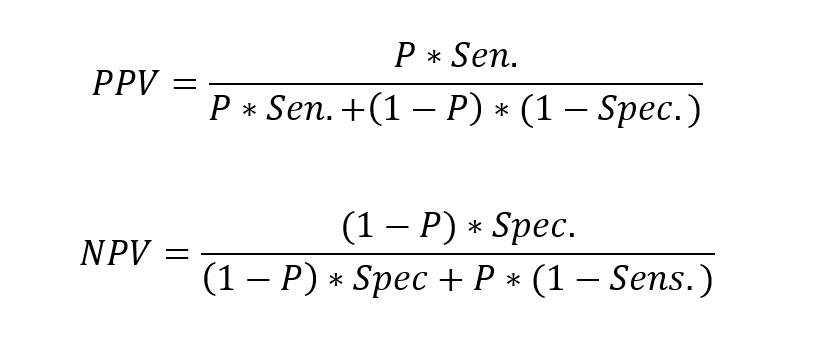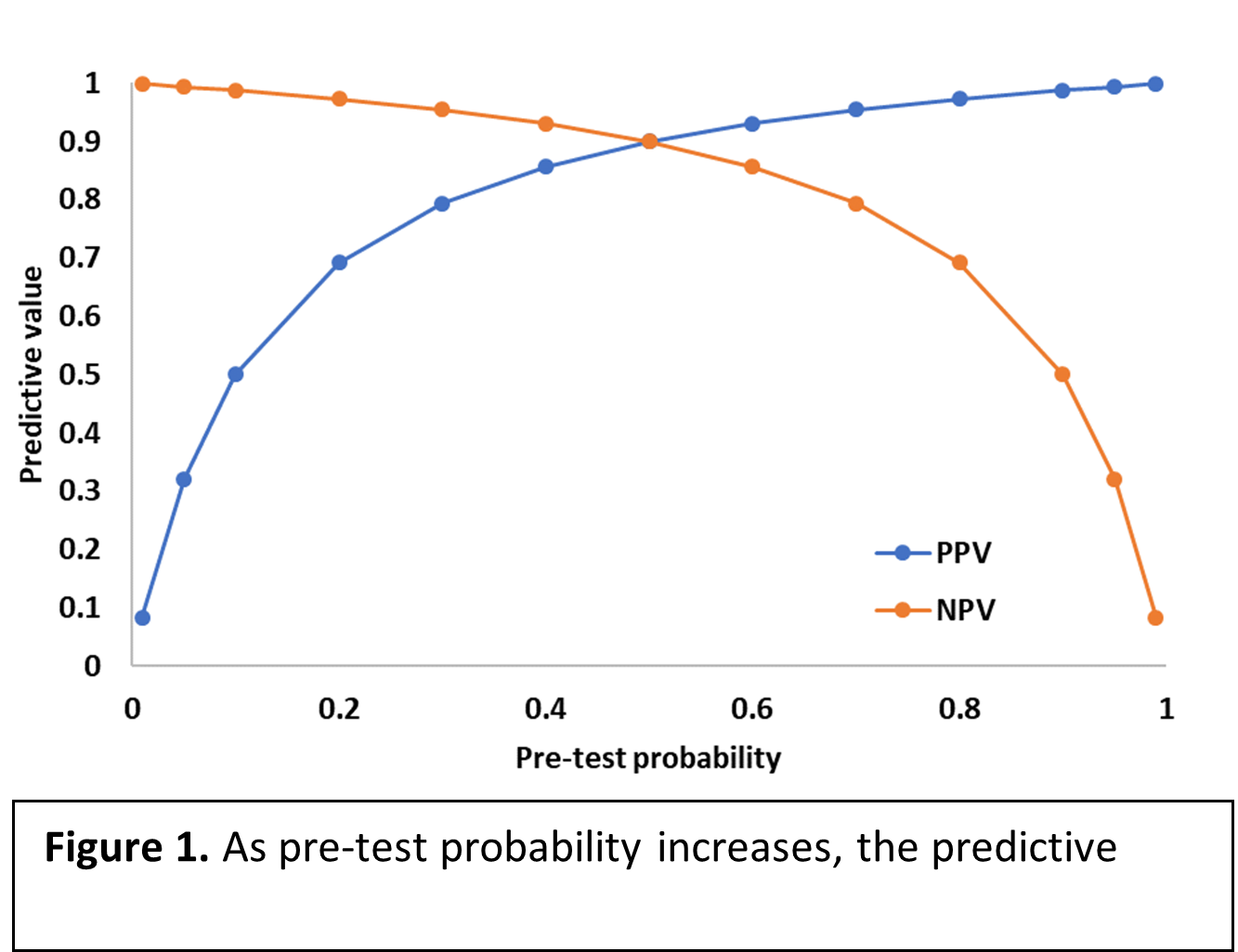# Predictive values

Diagnostic test performance is measured by sensitivity and specificity. However, neither of these measurements informs the practitioner on the probability of disease in individuals with positive or negative test results. The real question that practitioners face is given that a test result is positive, what is the probability that the individual actually has the disease or condition of interest? Predictive values help us answer this question.

Predictive values describe the probability that an observed test result reflects the disease-state of the individual tested. Predictive values are influenced by pre-test probability. Pre-test probability in the general population approximates the prevalence of the disease. However, in an individual patient, the history, clinical signs, etc. may influence disease probability to be greater or less than the general population. Therefore, the impact of disease prevalence on pre-test probability is considered to be the prevalence of disease in an imaginary population of animals identical to the individual in question. The formulas for predictive values using pre-test probability are as follows:As an example of the impact of pre-test probability on predictive value, if a heartworm test is performed on a stray dog in Mississippi with no history of heartworm prevention, the pre-test probability would describe the probability of heartworm disease in an identical population of dogs (i.e. stray dogs in Mississippi with no history of heartworm prevention). A positive heartworm test result for a dog from this population would be very believable (i.e. have a high predictive value) because the pre-test probability is very high. Figure 1 demonstrates that as pre-test probability increases, the predictive value of a positive test result increases as well.Similarly, when pre-test probability is low, the predictive value of a positive test result is low. The pre-test probability of heartworm disease in a stray dog in North Dakota would be much lower than the previously discussed pre-test probability in a stray dog in Mississippi because heartworm disease is rare in North Dakota.